# Adding to and Arranging Plots

We continue with the small bird data…

``````BirdData <- data.frame(
Tarsus  = c(22.3, 19.7, 20.8, 20.3, 20.8, 21.5, 20.6, 21.5),
Head    = c(31.2, 30.4, 30.6, 30.3, 30.3, 30.8, 32.5, 31.6),
Weight  = c(9.5, 13.8, 14.8, 15.2, 15.5, 15.6, 15.6, 15.7),
Wingcrd = c(59, 55, 53.5, 55, 52.5, 57.5, 53, 55),
Species = c('A', 'A', 'A', 'A', 'A',  'B', 'B', 'B')
)``````

# 1. Further details of `par()`

In the previous class, to modify elements of the graphic we added various arguments to the function `plot()`.

However, if you look at the help file (`?plot`), there are only three arguments specified: `plot(x, y, ...)`.

The argument `, ...` is special and allows arguments for one function to be passed through another function. We will use this explicitly when we write our own functions, but for now, we can see that arguments such as `cex =`, `pch =`, etc. are not used directly by `plot()`. These arguments are actually arguments for the graphical parameters function `par()`.

The parameters function, `par()`, is used to modify the basic appearance and layout of graphics.

There are a lot of parameters that you can control (see `?par`). Some you will use all the time, others will not be touched.

They are all listed on the `par()` help page. You should spend some time looking over this page to see what other options are available.

All the arguments listed below are placed within `par()`, e.g., `par(pch = 13, cex = 2)`.

Important! Once you use `par()` to change parameters, these settings will apply to all future graphics created in the open window

## Three ways to ‘reset’ `par()`:

1. Close the open graphics window. Any new window will have the default parameters.

2. Nest plotting within two further lines of code. Here, the graphic parameter settings are stored in the variable op, we make our figures using the modified parameters, and par(op) resets the parameters to their defaults.

``````op <- par(mfrow = c(2, 2), mar = c(3, 3, 2, 1))
plot( ... )
plot( ... )
par(op)``````
1. Create all figures in their own specific graphic device function, as described later.

# 2. Arranging plots

So far we have just placed one plot or panel in each graphics window. However, in many cases you will want multiple similar plots side-by-side, or different kinds of plots in the same overall figure.

There are various ways to do this.

Note: If you plot more figures than the number of panels available, they will start to overwrite from the beginning.

## Changing margin size

Margin sizes are specified in units of the number of lines of text.

### Setting the margins for individual plots: mar

`par(mar = c(5, 4, 4, 2))`

You can specify the amount of white space on each side of the graph

`par(mar = c(5, 4, 4, 2))` is the default, for sides clockwise from (bottom, left, top, right).

### Setting margins for the whole figure: oma

`par(oma = c() )`

A vector of the form c(bottom, left, top, right) giving the size of the outer margins in lines of text. This is useful to use in multi-panel plot (see below) where you need to add text to one side or another.

## Arranging multiple plots

You can establish a grid of panels which are then filled by separate calls to `plot()` (or another graphics plotting function).

### Regular arrangments

`par()` has two similar options, `mfrow =` and `mfcol =`.

Both arguments take a vector of the form `c(number of rows, number of columns)`. `mfcol =` fills this grid by colums, `mfrow =` fills by rows. e.g.:

• `par(mfrow = c(2, 2))` creates a graphics window with four panels, in a 2 x 2 layout.

• `par(mfcol = c(4, 1))` creates a column of four panels.

Here, we plot Head as a function of the four other variables in the data.

``````par(mfcol = c(2,2),
tcl = 0.5, mgp = c(2.5, 0.5, 0), las = 1
)

plot(Head ~ Tarsus, data = BirdData,
xlab = 'Tarsus (mm)',
pch = 20,
col = Species,
cex = 3)

plot(Head ~ Wingcrd, data = BirdData,
xlab = 'Wingcrd (m)',
pch = 20,
col = Species,
cex = 3)

plot(Head ~ Weight, data = BirdData,
xlab = 'Weight (kg)',
pch = 20,
col = Species,
cex = 3)

plot(Head ~ Species, data = BirdData,
xlab = 'Species',
pch = 20,
col = 1:2,
cex = 3)``````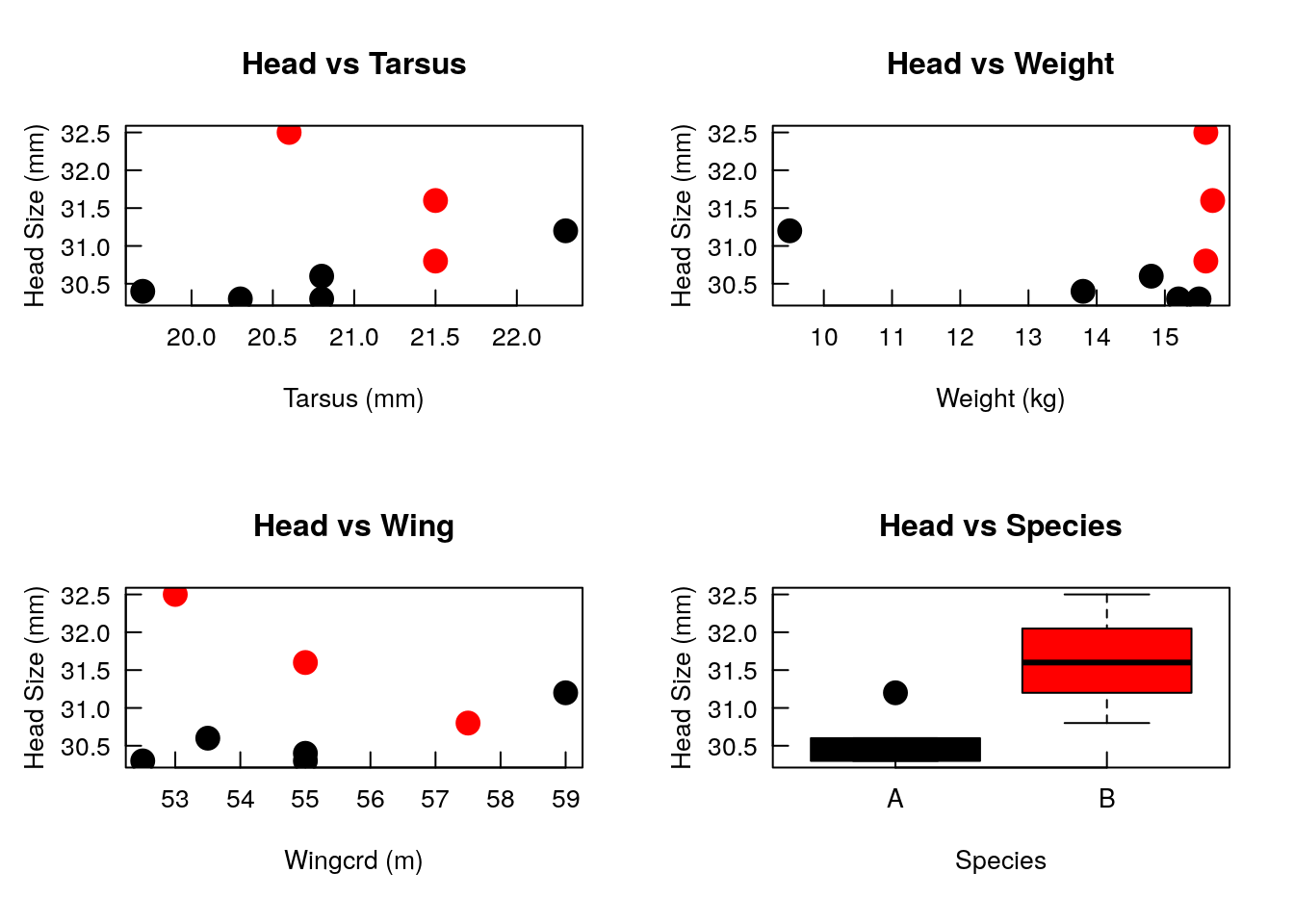### Irregular arrangements

The function `layout()` divides the plotting region/window up into as many rows and columns as there are in the argument `mat =` (a matrix), with the column-widths and the row-heights specified in the respective arguments.

Note that `layout()` is incompatible with `par(mfrow, mfcol)`.

This can be useful for setting up regular arrays, but more often it is used if you want different kinds of graphics together in the same figure.

For example, here, we plot the scatterplot of Head on Tarsus, but add a histogram of each to two sides.

``````# First, set up data for each histogram (we will cover this in more detail later)
# The plot = FALSE argument means that nothing is plotted,
# and the result is stored in the objects 'xhist' and 'yhist'
xhist <- hist(BirdData\$Head, plot = FALSE)
yhist <- hist( BirdData\$Tarsus, plot = FALSE)

# get maximum values
top <- max(c(xhist\$counts, yhist\$counts))

# set range of each data to match histograms to scatterplot axes
yrange <- c(30, 33)
xrange <- c(19.5, 22.5)

# use layout() to set up plotting region
nf <- layout(matrix(c(2,0,1,3),2,2,byrow = TRUE), c(3,1), c(1,3), TRUE)
layout.show(nf)``````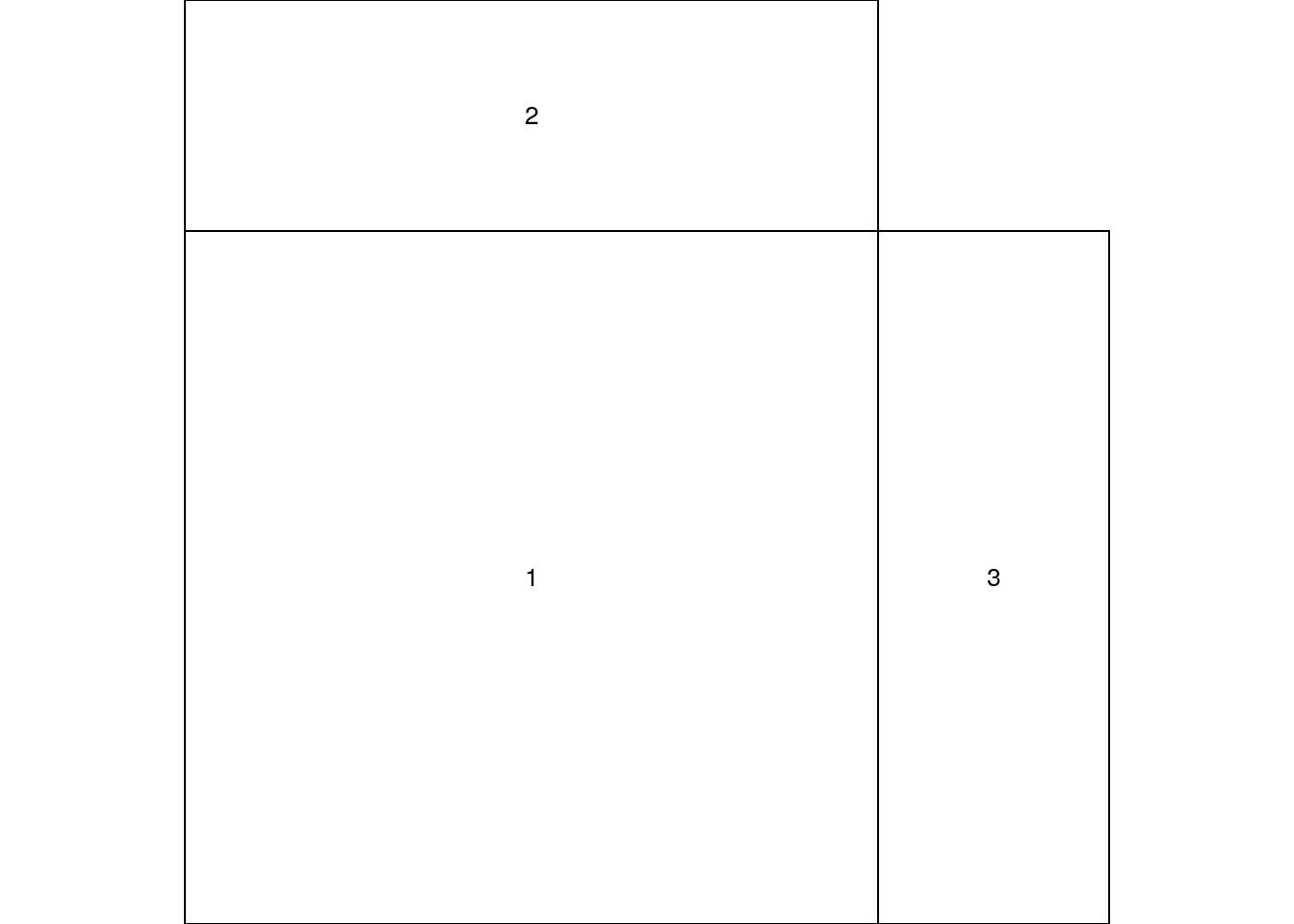``````# plot each part of the figure, setting margins to match up each time.
par(mar = c(5,5,1,1))
plot(Head ~ Tarsus, data = BirdData, xlim = xrange, ylim = yrange, xlab = "Tarsus (mm)", ylab = "Head Size (mm)")

par(mar = c(0,5,1,1))
barplot(xhist\$counts, axes = FALSE, ylim = c(0, top), space = 0)

par(mar = c(5,0,1,1))
barplot(yhist\$counts, axes = FALSE, xlim = c(0, top), space = 0, horiz = TRUE)``````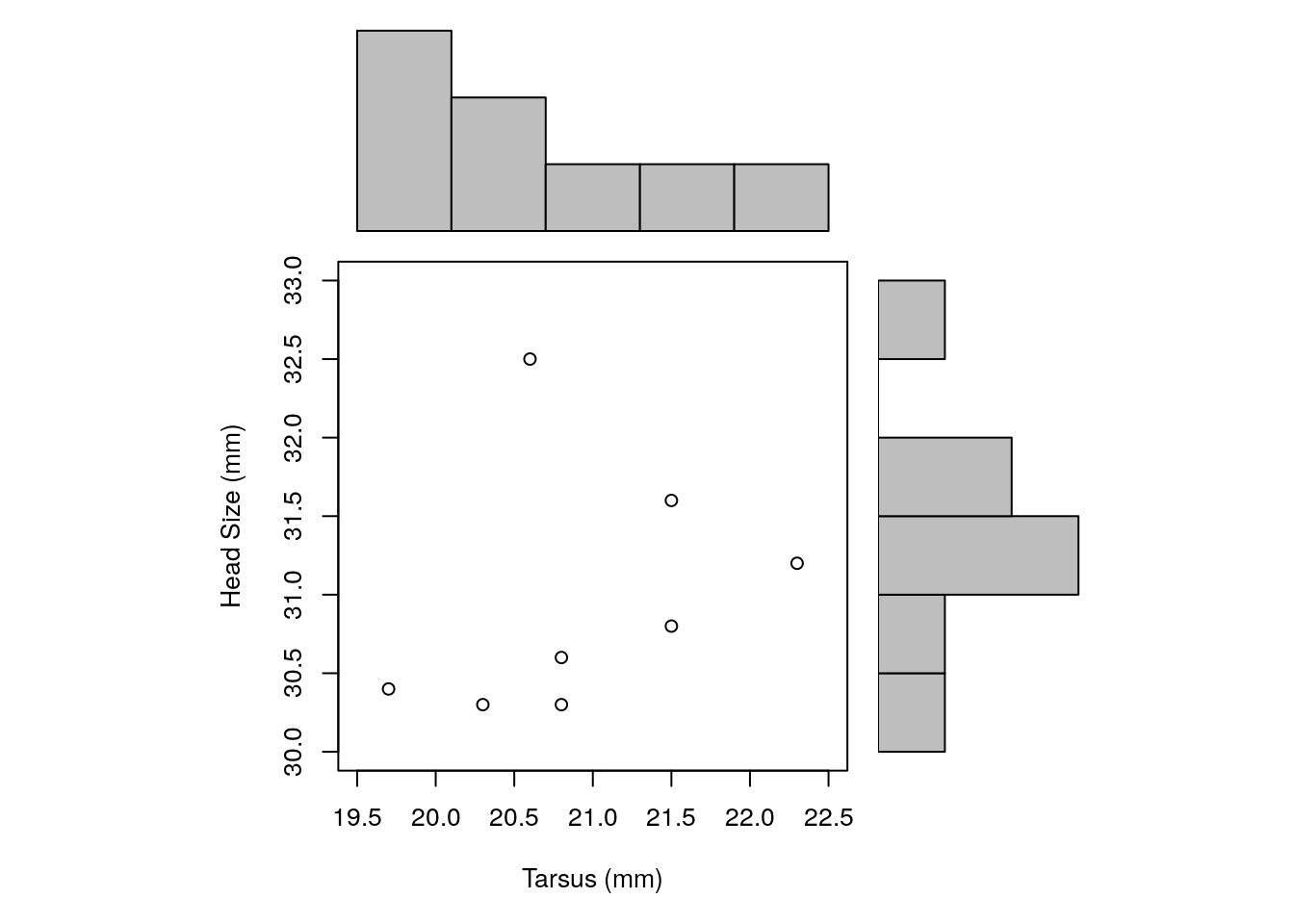In this example, we use `matrix()` to set up the order of plotting.

This command

``matrix(c(2,0,1,3), nrow = 2, ncol = 2, byrow = TRUE)``
``````##      [,1] [,2]
## [1,]    2    0
## [2,]    1    3``````

sets up a 2 x 2 matrix, where the first plot goes in the bottom left (1), second in top left (2), third in bottom right (3), with nothing in the top right (0).

The plan can be seen following the `layout.show()` command above.

# 3. Adding elements to a plot

All the subsequent commands are external to the functions `plot()` and `par()`.

They have to be run after you have created your plot.

## i. Text

### Text to the plot

`text(x = , y = , labels = )`

To add text within the plotting area, use `text()`.

This function places the text assigned to the argument `labels =` at the coordinates given by `x =` and `y =`.

• `x =`, `y =` numeric vectors of coordinates where the text ‘labels’ should be written.

• `labels =`a character vector or expression specifying the text to be.

• `, ...` further calls to `par()` to modify the text font, size, coluor, etc.

As usual with R, these three arguments can be vectors (and therefore columns in a dataframe).

Here, we can plot the species in place of the data points (remember that `type = ‘n’ stops plotting of the data).

``````plot(Head ~ Tarsus, data = BirdData, type = 'n')
text(x = BirdData\$Tarsus, y = BirdData\$Head, labels = BirdData\$Species, col = as.numeric(BirdData\$Species))``````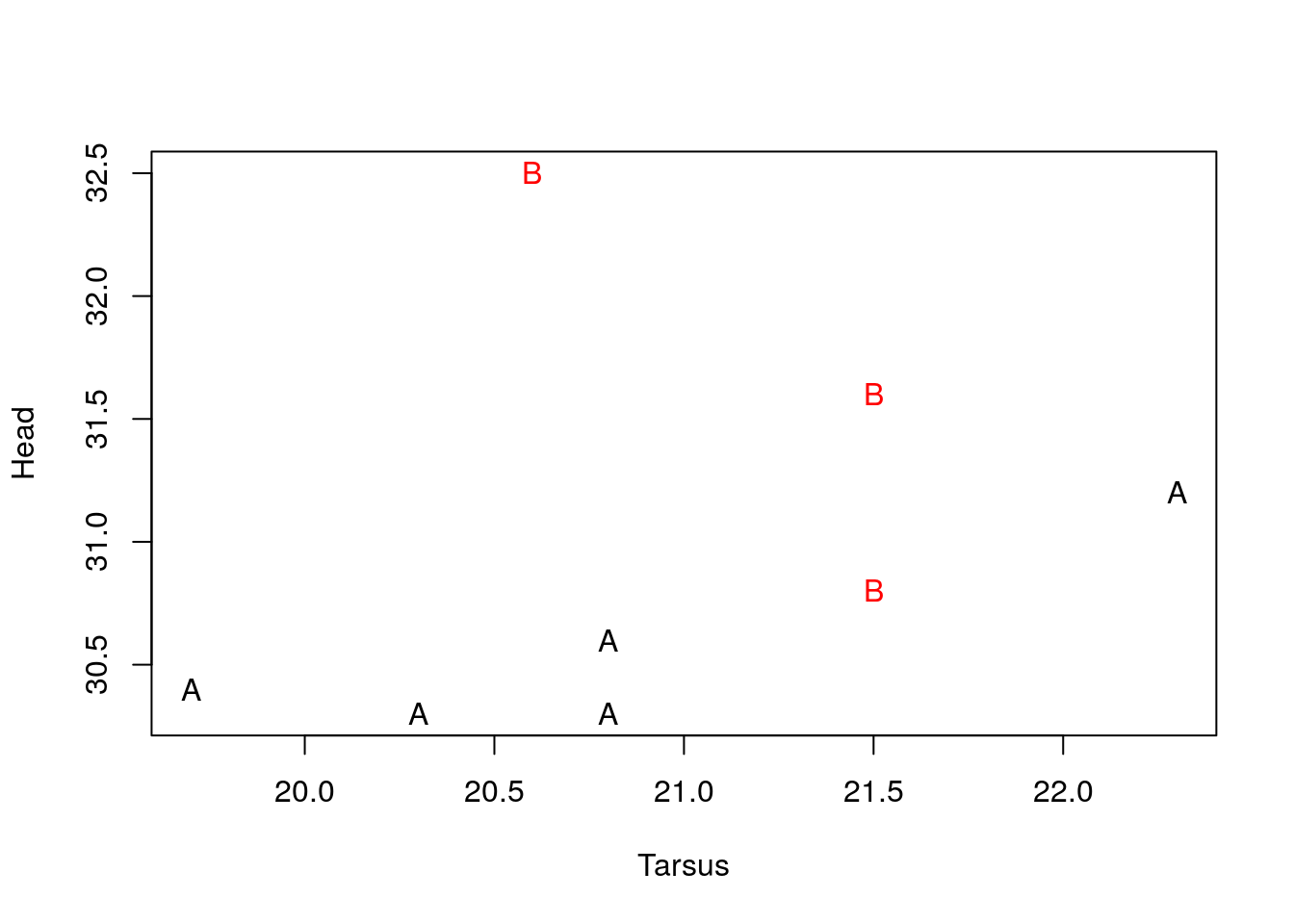To fine-tune the position of the text, use the following arguments:

• `adj =` one or two values in [0, 1] which specify the x (and optionally y) adjustment of the labels.

• `pos =` a position specifier for the text. If specified this overrides any ‘adj’ value given. Values of ‘1’, ‘2’, ‘3’ and ‘4’, respectively indicate positions below, to the left of, above and to the right of the specified coordinates.

### Text in the margin

`mtext(side = , line = , text = )`

The function `mtext()` adds text (e.g., axis labels) to any of the four margins.

• `side =` on which side of the plot (1 = bottom, 2 = left, 3 = top, 4 = right),

• `line =` the line number out from the plotting area, starting at 0 and counting outwards, where the text will print,

• `text =` a character string with the text to be printed.

### A legend

`legend(x = , y = NULL, legend = )`

The function `legend()` adds a legend to the current plot.

There are many arguments to this function, but the two main ones you will need are:

• `x =`, `y =` the location of the legend. You can either specify the coordinates of the top-left corner of the legend. Or, you can use `x =` a keyword from: ‘“bottomright”’, ‘“bottom”’, ‘“bottomleft”’, ‘“left”’, ‘“topleft”’, ‘“top”’, ‘“topright”’, ‘“right”’ and ‘“center”’.

• `legend =` a character or expression vector of length >= 1 to appear in the legend.

Once your legend is positioned, you will need to use one or more arguments from `par()` to match the symbols/lines with what you have plotted in the figure, e.g., `pch =`, `col =`, `lty =`.

``````plot(Head ~ Tarsus, data = BirdData, pch = as.numeric(Species))
legend(x = 'topright', legend = levels(BirdData\$Species),
pch = unique(as.numeric(BirdData\$Species) ))``````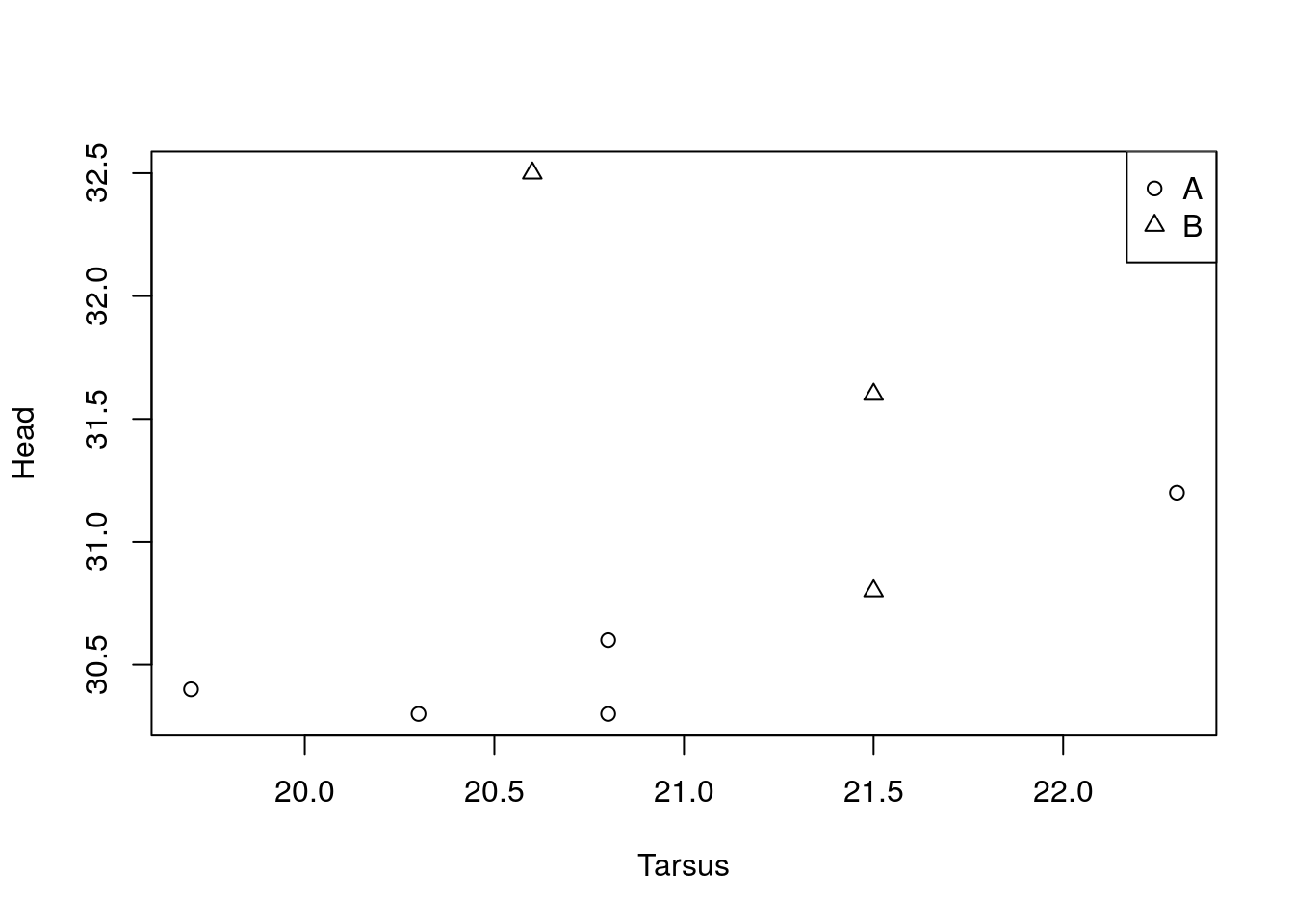## ii. Points

`points(x = , y = , type = 'p', pch = , ...)`

Like `text()` and `lines()`, points requires coordinates and what you want to plot there.

Further, `points()` and `lines()` can be used interchangeably, given that you can use the `type =` argument to switch between points and lines.

• `x =`, `y =` coordinate vectors of points to plot.

• `type = 'p'` character indicating the type of plotting. Remember, this is the same argument from `plot()`: ‘p’ = points, ‘l’ = line, ‘b’ = both.

• `pch =` set the plotting symbol.

• `col =` set plotting symbol colour.

Modify the point appearance with further calls to arguments of `par()`, within the call to `points()`.

``````plot(Head ~ Tarsus, data = BirdData, type = 'n')
points(x = BirdData\$Tarsus, y = BirdData\$Head,
pch = as.numeric(BirdData\$Species),
col = as.numeric(BirdData\$Species),
lwd = 2)``````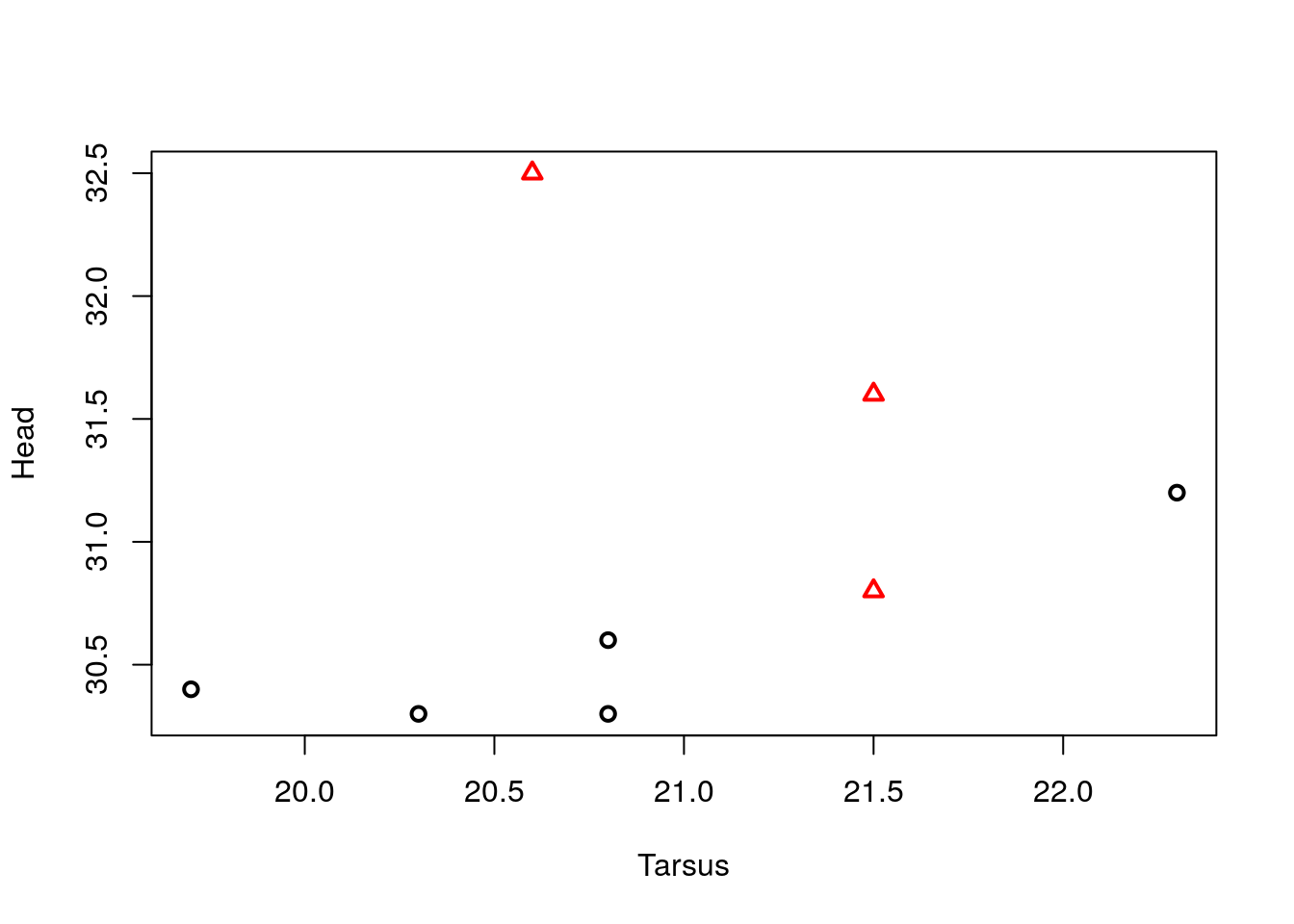## iii. Lines

There are several functions for adding lines to a plot, from a single straight line (`abline()`), to connecting multiple points (`lines()`), to adding sets of small straight segments (`segments()` or `arrows()`).

`abline(a = NULL, b = NULL, h = NULL, v = NULL, reg = NULL, coef = NULL, ...)`

The function `abline()` plots a straight line across the whole plotting area, often extending beyond the range of the data you have.

Notice that all the arguments have a default of NULL. Thus, this function can work with a variety of different inputs very easily.

We have used `abline()` previously to work with a model output:

• `reg =` an object with a ‘coef’ method. `abline()` calls `coef()` to extract the intercept and line from a call to `lm()` and plot the line.

We can also provide these two values directly:

• `coef =` a vector of length two giving the intercept and slope,

or with

• `a =`, `b =` an intercept and slope.

Another useful feature of `abline()` is the ability to plot straight horizontal or vertical lines (e.g., if you want to plot a line at 0, or some other specific value(s)).

• `h =` the y-value(s) for a horizontal line(s),

• `v =` the x-value(s) for vertical line(s).

Again, calls to the arguments of `par()` can be used to change the appearance of these lines.

``````plot(Head ~ Tarsus, data = BirdData)
abline(v = 21, lwd = 2, lty = 3)``````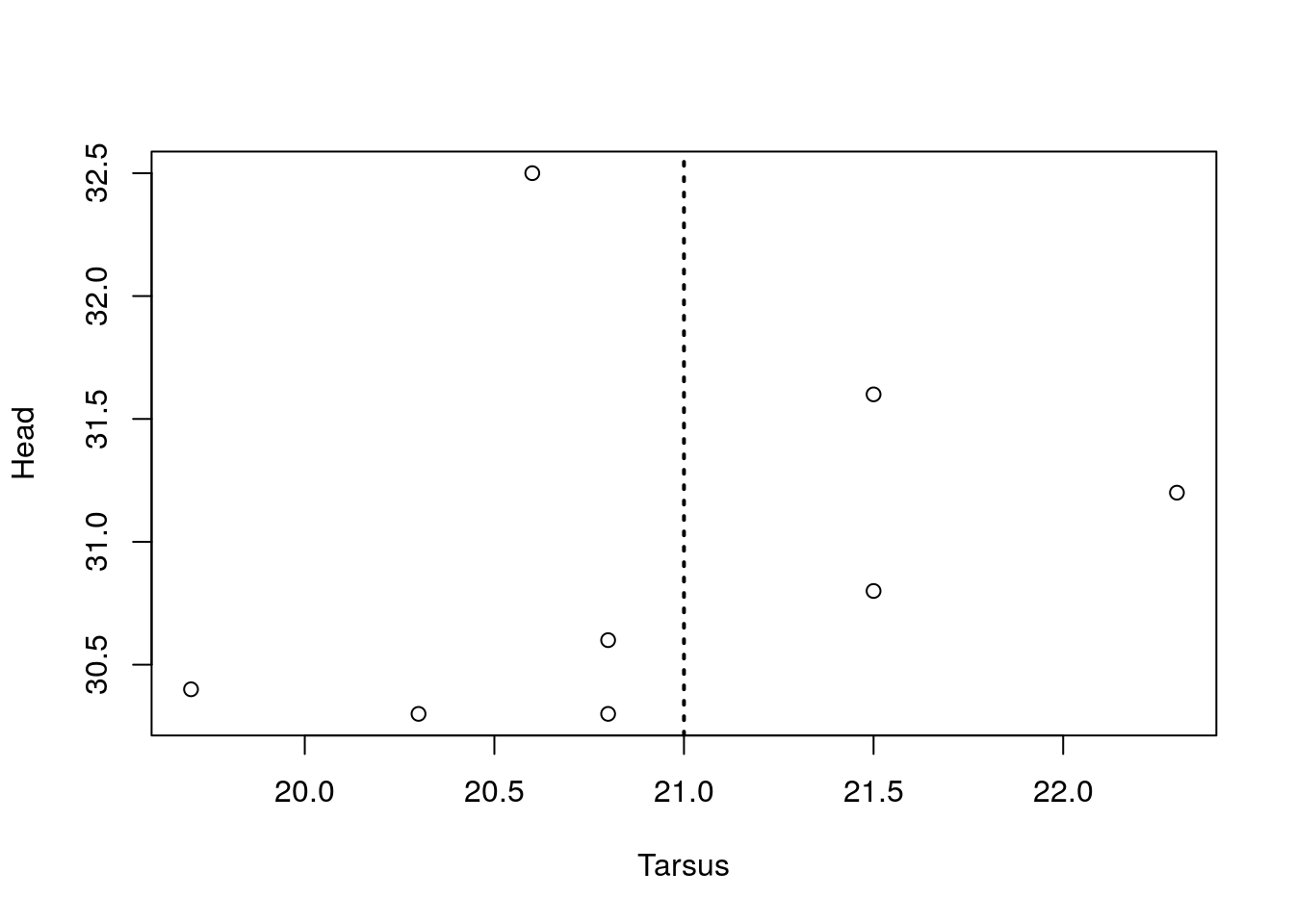### Add any kind of (fitted) line

`lines(x = , y = , type = 'l', ...)`

Like `text()` and `points()`, `lines()` requires coordinates and what you want to plot there.

Further, `points()` and `lines()` can be used interchangeably, given that you can use the `type =` argument to switch between points and lines.

• `x =`, `y =` coordinate vectors of points to plot.

• `type = 'p'` character indicating the type of plotting. Remember, this is the same argument from `plot()`: ‘p’ = points, ‘l’ = line, ‘b’ = both.

Recall that we can use many arguments from `par()` to change the appearance of a line:

• `lty =` the line type: 0 = blank, 1 = solid (default), 2 = dashed, 3 = dotted, 4 = dotdash, 5 = longdash, 6 = twodash.

• `lwd =` the line width,

• `col =` line colour.

There are many instances where you may want to join a line between two points. This could be to present error bars around your data points, or indicate that two points are related in some way (below).

For error bars, you can use the functions `arrows()` or `segments()`.

Both work in the same way, but `arrows()` provides a ‘cap’ (or, indeed, an arrow) to the end of the line, whereas `segments()` does not.

`arrows(x0, y0, x1 = x0, y1 = y0, length = 0.25, angle = 30, ...)`

`segments(x0, y0, x1 = x0, y1 = y0, ...)`

• `x0 =`, `y0 =` coordinates of points from which to draw.

• `x1 =`, `y1 =` coordinates of points to which to draw. At least one must be supplied.

• `col =`, `lty =`, `lwd =` graphical parameters as in ‘par()’, possibly vectors.

For each x,y coordinate (i.e., each ‘i’), a line segment is drawn between the point ‘(x0[i], y0[i])’ and the point ‘(x1[i], y1[i])’.

To plot a horizontal arrow head, the default angle needs to be changed to 90.

In this case we are plotting two error bars at a time.

``````## calculate mean and sd values with tapply()

plot(y = yv0, x = 1:2,
ylim = c(26, 34), xlim = c(0.5, 2.5),
cex = 2, lwd = 2)

## top error bar
arrows(x0 = 1:2, y0 = yv0, # arrow from ...
x1 = 1:2, y1 = yv0 + yv1, # arrow to ...
lwd = 2, angle = 90, length = 0.2)

## bottom error bar
arrows(x0 = 1:2, y0 = yv0, # arrow from ...
x1 = 1:2, y1 = yv0 - yv1, # arrow to ...
lwd = 2, angle = 90, length = 0.2)``````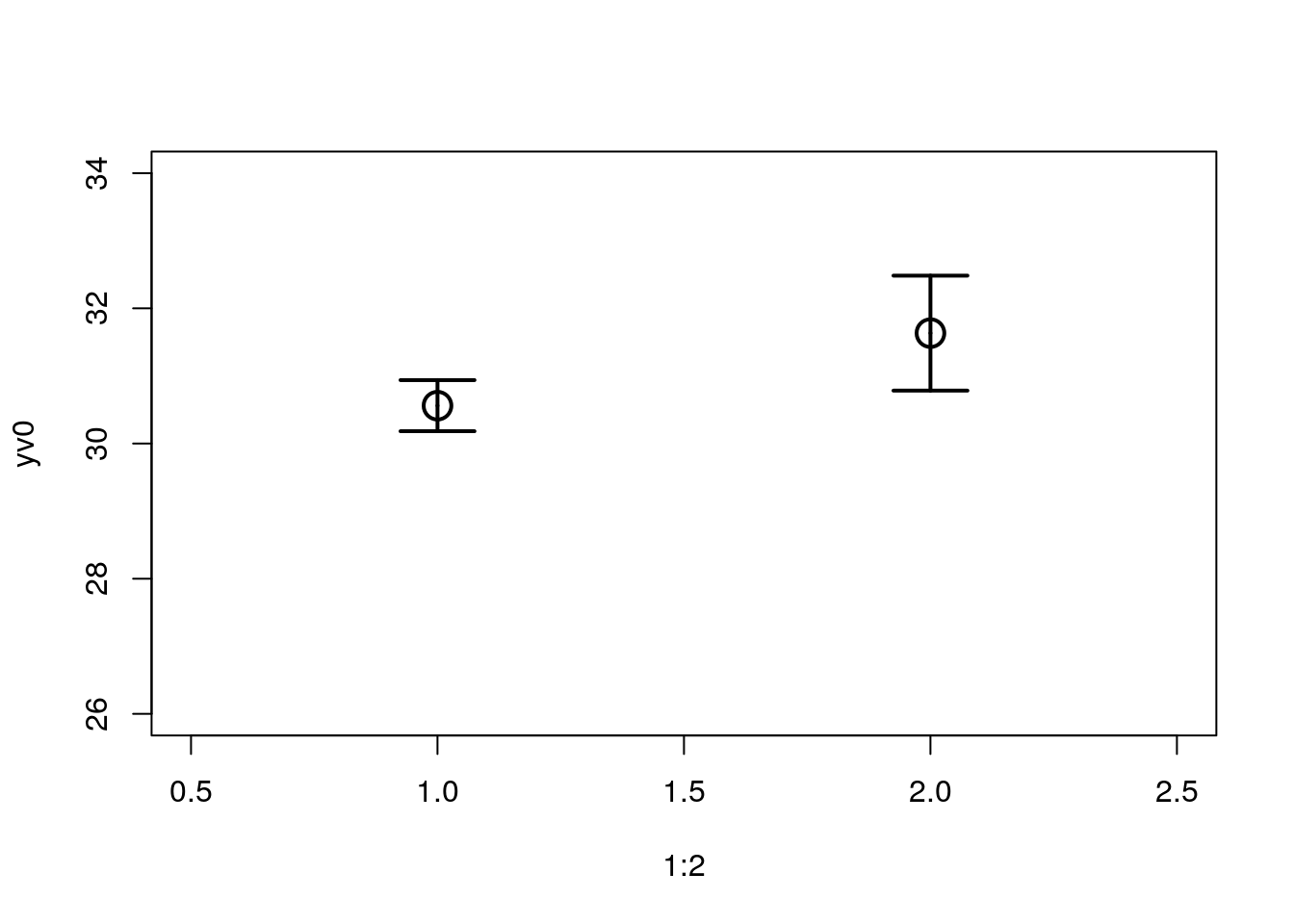### Join two points

`segments(x0, y0, x1 = x0, y1 = y0, ...)`

We can use the function `segments()` to link two points.

Here we plot just one line.

``````## calculate mean and sd values with tapply()

plot(y = yv0, x = 1:2,
ylim = c(26, 34), xlim = c(0.5, 2.5),
cex = 2, lwd = 2)

segments(x0 = 1, y0 = yv0, # line from ...
x1 = 2, y1 = yv0, # line to ...
lwd = 2, lty = 2, col = 'grey')``````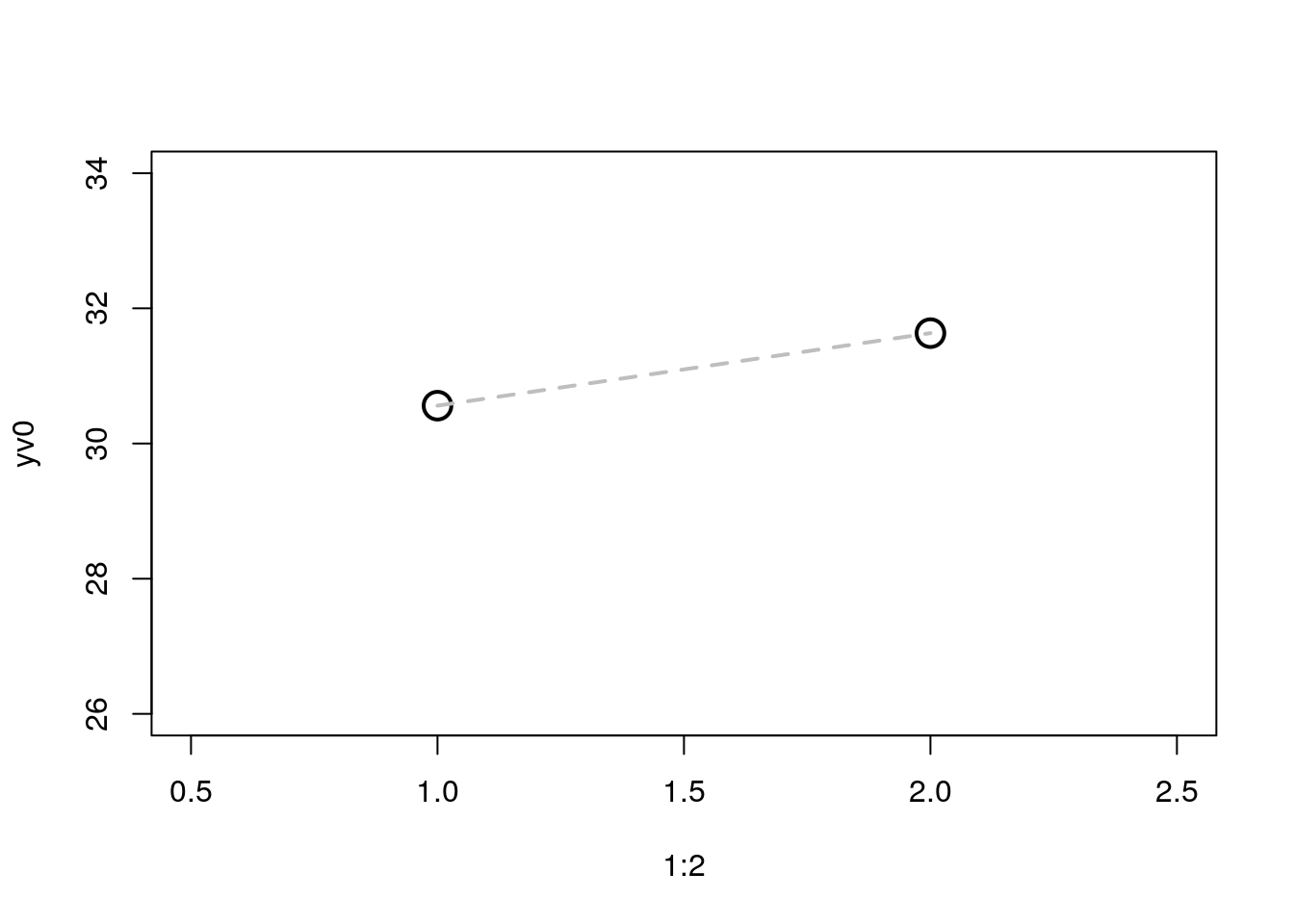## iv. Axes

Here, you have to specify which side of the plot and where you want the tick marks and their labels.

`axis(side = , at = , labels = , ...)`

• `side =` an integer specifying which side of the plot the axis is to be drawn on. The axis is placed as follows: 1=below, 2=left, 3=above and 4=right.

• `at =` the points at which tick-marks are to be drawn.

• `labels =` this can either be a logical value specifying whether (numerical) annotations are to be made at the tickmarks, or a character or expression vector of labels to be placed at the tickpoints.

``````## calculate mean and sd values with tapply()